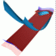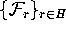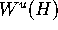Abstract and Contents
Up: 3. The algorithm
Next: 3.2 Globalizing by adding discrete circles
Previous: 3. The algorithm

# 3.1 Iterating a fundamental domain

The standard approach for globalizing one-dimensional unstable manifolds is to start with a point on the linear approximation, take the line segment between this point and its f-image, and compute iterates of this (approximation of a) fundamental domain. For the two-dimensional version of this method, one would take a circle of points on the linear approximation. If the circle is close enough to H, one may assume that the f-image of this circle is almost a circle as well. Therefore, one obtains an annulus as a fundamental domain.

Since the fundamental domain cannot be iterated as a continuous object, one needs to discretize it. As was pointed out earlier, merely iterating the points of the discretiation will lead to a possible accumulation of points on a one-dimensional submanifold of; see also Figure 2. In our situation, we can avoid this accumulation by keeping track of where the iterates of the circle intersect. However, there is a second problem. Away from H, the iterates of the initial circle on the linear approximation ofmay not look like circles at all. In practice, already after a few iterations, the successive images of the fundamental domain may have a very `unpractical' shape. For example in the 3D-fattened Arnold family in Section 5.1, the invariant circle contains two fixed points. Close to the one-dimensional stable manifold of the fixed point that is repelling on H, the f-image of an annulus grows a `nose' that stretches and winds around the circle several times, but at least once. This is unpractical, because it is unclear how one can obtain a nice mesh on the unstable manifold in this situation. It may look like the unstable manifold has holes.

In order to avoid such problems we drop the idea of iterating a fundamental domain. Instead we let the manifold grow at the same speed in each direction, which means that we add rings of fixed width. How this can be done is explained in the following section.

Up: 3. The algorithm
Next: 3.2 Globalizing by adding discrete circles
Previous: 3. The algorithmAbstract and Contents

Written by: Bernd Krauskopf & Hinke Osinga
Created: May 27 1997 --- Last modified: Fri May 30 19:49:51 1997